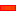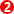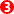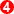﻿ Hypotrochoid

# Hypotrochoid

## Hypocycloid and Hypotrochoid Curves

####Hypocycloid and Hypotrochoid Curves

The Hypotrochoid Curves are produced by a Point on a gear that rolls (orbits) around the inside of the fixed gear.

There are two sets of Hypotrochoid Curves.

 • The Stationary Gear has inward facing teeth with the revolving gear orbiting inside.
 • The Stationary Gear has outward facing teeth with the revolving gear orbiting outside. These curves are sometimes called Peritrochoid Curves. See Peritrochoid Curves

This topic will focus on Hypotrochoid Curves.

The shape of the Hypotrochoid curve depends on the ratio of the teeth. With gears, the ratio of the teeth is always a rational number. Therefore, the curve will always repeat eventually even if the gear ratio is a ratio of two prime numbers, for example.### The Revolving Gear is half the diameter of the fixed gear..

The revolving gear is inside, and is half the diameter of the fixed outside gear.

There are four curves

One curveis given by a point on the pitch-circle - it is the Hypocycloid

In this case, when the ratio of teeth is 1:2, it is a straight line.

Hypotrochoid Curves

In this case the three other curves are ellipses.

Two curvesare given by points outside the pitch-circle

One curveis given by a point inside the pitch-circleThe revolving gear inside, is 2.3 the diameter of the fixed outside gear.

Hypoycloid

One curve is given by a point on the pitch-circle - it is the Hypocycloid

Hypotrochoid

Two curves are given by points outside the pitch-circle

You can see the curve is approximately a square.

You can use the Euler-Savary Equation to calculate the best distance from the centre of the orbiting gear to give a curve with the best 'straight-line' curve.Gear Ratio is 48:18
There is ONE Curve.
You must rotate the carrier 3 times to complete the Hypocycloid Path.

Hypocycloid

One curve is given by a point on the pitch-circle - it is the Hypocycloid

The Gear Ratio is 48:18.

Tutorials and Reference Help Files for MechDesigner and MotionDesigner 14.2 + © Machine, Cam, Mechanism, and Motion Design Software by PSMotion Ltd